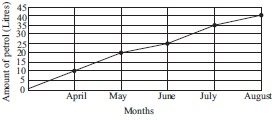# User ForumSubject :IMO    Class : Class 5

Study the given line graph which shows the capacity of petrol Mohit used while travelling from City X to City Y from April to August and answer the following questions.(A) How much more capacity of petrol was used in August than in May?
(B) What fraction of the total capacity of petrol used in July?

 (A) (B) A 20000 mL 7/26 B 22000 mL 8/29 C 10000 mL 7/27 D 25000 mL 9/36

Ans not understood... I doubt if the ans is correct.

Class : Class 6
Option A)

## Ans 2:

Class : Class 6
Ans is A because the answer of question (A) will be 20000 ml because 40 l-20 l=20 l. Now convert 20 l to ml, which is 20000 ml, or, you can say that 20 l and 40 l will be 20000 ml and 40000 ml, respectively. Now do 20000 ml-40000 ml=20000ml. answer of question (B) will be 7/26 by adding the capacity of petrol of April, May, June, July and August, which will be 10 l+20 l+ 25 l+35 l+40 l=130 l. In July, the capacity of petrol used was 35 l, so in fraction, it will be 35 l/130 l. Now simplify the fraction, which will be 7 l/26 l.